• np.random.shuffle函数
2020-04-17 17:20:55

## 代码：

np.random.shuffle(x_data)

x_data是一个张量，该函数用于将x——data自身打乱，注意是在原数组上进行的，不是返回一个新数组，而原张量不变。

更多相关内容
• 注：使用方法与np.random.randn()函数相同 作用： 通过本函数可以返回一个或一组服从“0~1”均匀分布的随机样本值。随机样本取值范围是[0,1)，不包括1。 应用：在深度学习的Dropout正则化方法中，可
• np.random.shuffle(index) print(index[0:20]) X_train=X_train[index,:,:,:]#X_train是训练集，y_train是训练标签 y_train=y_train[index] 补充知识：Keras中shuffle和validation_split的顺序 模型的fit函数有两个...
• numpy.random.shuffle 在做将caffe模型和预训练的参数转化为tensorflow的模型和预训练的参数，以便微调，遇到如下函数： def gen_data(source): while True: indices = range(len(source.images)) # indices = ...
• np.random.shuffle(x) ：在原数组上进行，改变自身序列，无返回值。 np.random…permutation(x) ：不在原数组上进行，返回新的数组，不改变自身数组。 1. np.random.shuffle(x) 1. 对一维数组重新排序： import ...
• 对给定的数组进行重新排列的方式主要有两种：
1. np.random.shuffle(x) ：在原数组上进行，改变自身序列，无返回值
2. np.random…permutation(x) ：不在原数组上进行，返回新的数组，不改变自身数组

## 1. np.random.shuffle(x)

#### 1. 对一维数组重新排序：

import numpy as np

arr = np.arange(10)
print(arr)

np.random.shuffle(arr)
print(arr)#### 2. 对多维数组重新排序：

arr = np.arange(12).reshape(3,4)
print(arr)

np.random.shuffle(arr)
print(arr)

np.random.shuffle(arr)
print(arr)通过上述例子可以看出，对于一个多维的输入，只是在第一维上进行了随机排序。例如对这个3×4的矩阵来说，只是对行进行随机排序。

## 2. np.random.permutation(x)

#### 1. 可直接生成一个随机排列的数组：

np.random.permutation(10)#### 2. 对一维数组随机排序：

>>> import numpy as np
>>> a=np.random.permutation([0,1,2,3,4,5,6,7,8,9])
>>> print(a)#### 3. 对多维数组随机排序：

arr = np.arange(9).reshape((3, 3))
print(arr)

arr2 = np.random.permutation(arr)
print(arr)
print(arr2)通过上述例子可以看出，对于一个多维的输入，只是在第一维上进行了随机排序。例如对这个3×3的矩阵来说，只是对行进行随机排序。

## 3. np.random.shuffle(x) 与 np.random.permutation(x) 的区别：

• 从代码可以看出，两个函数的功能类似。np.random.shuffle(x)改变自身数组，np.random.permutation(x)不改变自身数组。
展开全文python numpy
• Permutation：（一组事物可能的一种） 序列，排列，排列中的任一组数字或文字; 这个函数的使用来随机排列一个数组的，第一个例子如图1所示： ...permutation = list(np.random.permutation(m)) #m为样本数 s

Permutation：（一组事物可能的一种） 序列，排列，排列中的任一组数字或文字;
这个函数的使用来随机排列一个数组的，第一个例子如图1所示：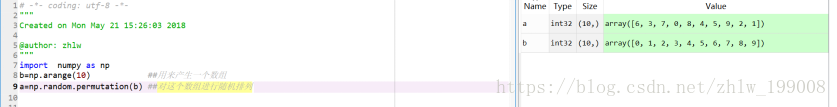对多维数组来说，是多维随机打乱而不是1维，例如：
第一次运行结果（代码在左侧），如图2所示：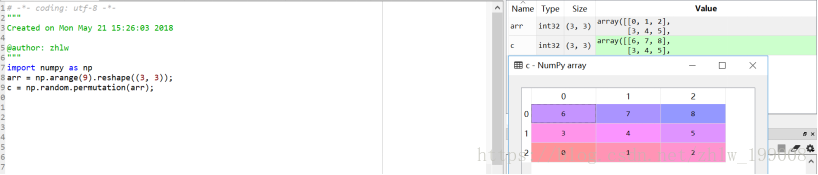第二次运行结果（代码在左侧），如图3所示：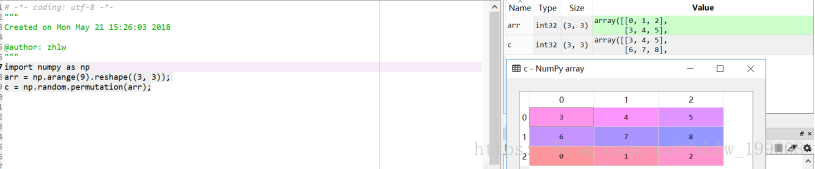如果要利用次函数对输入数据X、Y进行随机排序，且要求随机排序后的X Y中的值保持原来的对应关系，可以这样处理：
permutation = list(np.random.permutation(m)) #m为样本数
shuffled_X = X[permutation]
shuffled_Y = Y[permutation].reshape((1,m))
图4中的代码是针对一维数组来说的,(图片中右侧为运行结果)：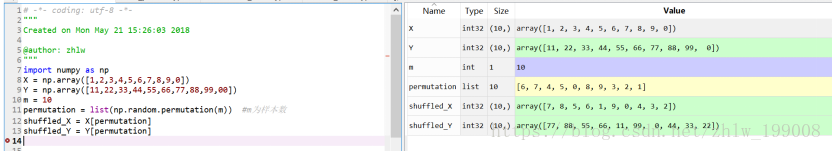图5中的代码是针对二维数组来说的，(图片中右侧为运行结果)：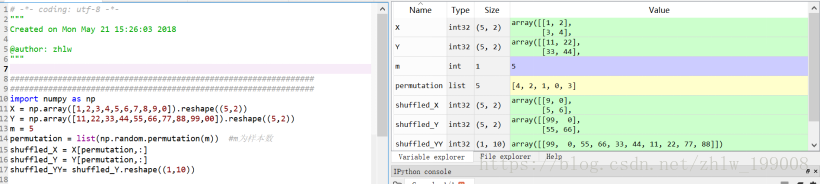代码示例：

# permutation()函数使用示例
def testPermutation():
print("==================打乱数组元素的顺序==================")
x = np.arange(10).reshape(5, 2)
print("原数组：")
print(x)
x_permutation = np.random.permutation(x)
print("打乱后的数组：")
print(x_permutation)

print("\n==================对应打乱2个数组元素的顺序==================")
print("原数组：")
x = np.array([0, 1, 2, 3, 4, 5, 6, 7, 8, 9])
y = np.array([1, 0, 1, 0, 1, 0, 1, 0, 1, 0])
print(x)
print(y)

m = 10 # 元素个数
permutation_array = np.random.permutation(m) # <class 'numpy.ndarray'> [0 7 3 2 1 8 4 6 5 9]
permutation = list(permutation_array) # [0, 7, 3, 2, 1, 8, 4, 6, 5, 9]

shuffled_X = x[permutation]
shuffled_Y = y[permutation]
print("打乱后的数组：")
print(shuffled_X) # [0 7 3 2 1 8 4 6 5 9]
print(shuffled_Y) # [1 0 0 1 0 1 1 1 0 0]



运行结果：

**加粗样式**==================打乱数组元素的顺序==================
原数组：
[[0 1]
[2 3]
[4 5]
[6 7]
[8 9]]
打乱后的数组：
[[8 9]
[2 3]
[4 5]
[6 7]
[0 1]]

==================对应打乱2个数组元素的顺序==================
原数组：
[0 1 2 3 4 5 6 7 8 9]
[1 0 1 0 1 0 1 0 1 0]
打乱后的数组：
[5 7 4 9 8 0 3 1 2 6]
[0 0 1 0 1 1 0 0 1 1]



shuffle()函数使用示例：
shuffle() 方法将序列的所有元素随机排序

# shuffle函数使用示例：
def testShuffle():
list = [20, 16, 10, 5]
np.random.seed(12)
np.random.shuffle(list)
print("随机排序列表 : ", list)
np.random.seed(12)
np.random.shuffle(list)
print("随机排序列表 : ", list)

**运行结果：**

随机排序列表 :  [20, 16, 10, 5]
随机排序列表 :  [20, 16, 10, 5]

展开全文python 排序算法 算法
• ## numpy的np.random.shuffle

万次阅读 多人点赞 2020-02-26 12:35:28
np.random,shuffle作用就是重新排序返回一个随机序列作用类似洗牌，我自己也写过一个洗牌小程序 下图是我做的一个尝试

np.random,shuffle作用就是重新排序返回一个随机序列作用类似洗牌，我自己也写过一个洗牌小程序
下图是我做的一个尝试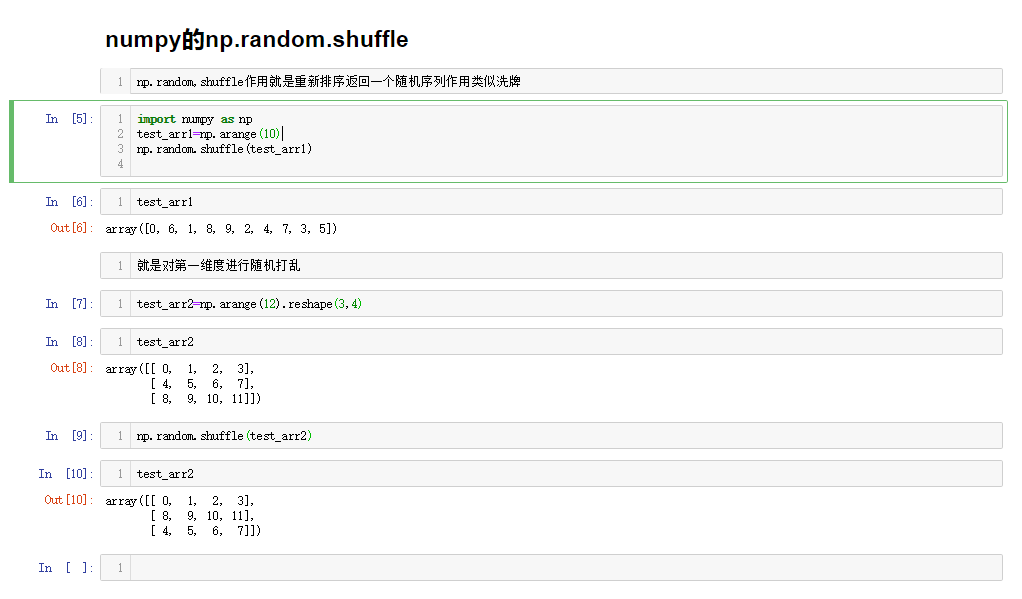展开全文• 功能：生成随机列表 x = np.arange(10) print(x) np.random.shuffle(x) print(x) 输出： [0 1 2 3 4 5 6 7 8 9] [2 1 8 4 3 5 0 9 7 6]
• 主要介绍了python numpy之np.random的随机数函数使用介绍,需要的朋友可以参考下
• np.random random() 生成一个[0, 1.)之间的随机浮点数 random([3, 2]) 生成形状为3×2的[0, 1.)之间的随机浮点数 rand(3, 2) 和random([3, 2])相同 randint(a ,b, size) 生成[a,b)之间的随机整数...python
• np.random.shuffle(): 洗牌，生成随机列表，打乱原有的顺序 import random import numpy as np data = np.arange(6) print(data) np.random.shuffle(data) print(data) 输出结果： [0 1 2 3 4 5] [2 3 4 5 0 1]python 机器学习
• 函数shuffle与permutation都是对原来的数组进行重新洗牌（即随机打乱原来的元素顺序）。区别在于shuffle直接在原来的数组上进行操作，改变原来数组的顺序，无返回值。而permutation不直接在原来的数组上进行操作，...
• 1.np.random.random()函数参数 np.random.random((1000, 20)) 上面这个就代表生成1000行 20列的浮点数，浮点数都是从0-1中随机。 2.numpy.random.rand()函数用法 numpy.random.rand(d0, d1, ..., dn)： 生成一个[0...
• 当你将seed值设为某一定值，则np.random下随机数生成函数生成的随机数永远是不变的。更清晰的说，即当你把设置为seed(0)，则你每次运行代码第一次用np.random.rand()产生的随机数永远是0.5488135039273248；第二次用...numpy Python
• ## np.random.shuffle

千次阅读 2019-04-19 11:54:45
https://blog.csdn.net/lyy14011305/article/details/76207327
• 描述： shuffle() 方法将序列的所有元素随机排序。 #实验可得每次shuffle后数据都被打乱，这个方法可以在机器学习训练 #的时候在每个epoch结束后将数据重新洗牌进入下一个epoch的学习 ...np.random.shuf
• 最近进行建模时，需要经常自己打乱数据集进行，numpy提供了一个函数可以对已有列表进行打乱 可以很方便的根据ID自己划分数据，当然为了使得每次划分数据相同，最好自己设定numy的随机种子 numpy.random.seed(num...
• np.random函数的几个方法1. randint方法2. random_integers方法3. randn方法4. random方法5. rand方法6. choice方法7. shuffle方法8. permutation方法9. seed方法10. RandomState方法11. uniform方法12. 等同于...python numpy
• 1.np.random.random()函数参数 np.random.random((1000, 20)) 上面这个就代表一千个浮点数，从0-20中随机。 2.numpy.random.rand()函数用法 numpy.random.rand(d0, d1, ..., dn) 生成一个[0,1)之间的随机浮点数或N...
• np.random.rand()与np.random.randn()函数用法相同 np.random.rand()函数 作用： 返回一个或一组服从“0~1”均匀分布的随机样本值。随机样本取值范围是[0,1)，不包括1。 import numpy as np print(np.random..python
• ...1.np.random.random()函数参数 np.random.random((1000, 20)) 上面这个就代表生成1000行 20列的浮点数，浮点数都是从0-1中随机。 2.numpy.random.rand()函数用法 numpy.rando...
• 在进行机器学习和深度学习中，我们会经常用到np.random.seed()，利用随机数种子，使得每次生成的随机数相同。 numpy.randn.randn(d0，d1，...，dn) randn函数根据给定维度生成大概率在（-2.58~+2.58）之间的数据 ...python 开发语言 后端
• 1python自带的random库 随机生成一个n-m之间的整数： random.randint(1,10) 随机生成一个浮点数： random.random()，什么参数都不需要给，随机生成一个0到1之间的浮点数； random.uniform(1.1,5.4)，随机生成一个...python pycharm 开发语言
• ## numpy.random.shuffle打乱顺序函数

万次阅读 多人点赞 2016-12-29 22:05:59numpy...